# How to read an imperial micrometer?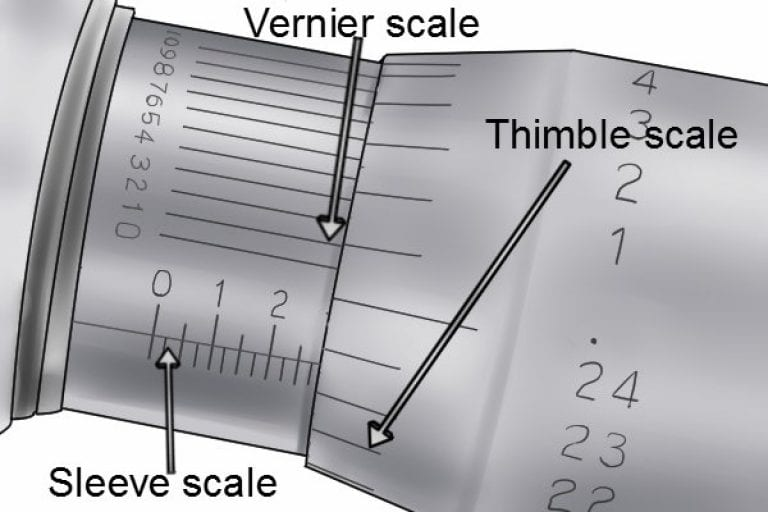Measurements taken with a micrometer are a combination of the value shown on the sleeve scale and the value indicated on the rotating sleeve scale.

When using a micrometer with an additional vernier scale, the value on the vernier scale may also be taken into account.

## Guide to Reading an Imperial Micrometer

### Step 1 - Look at the sleeve scale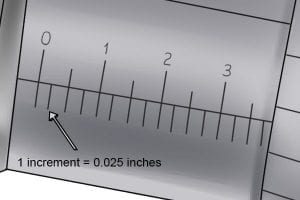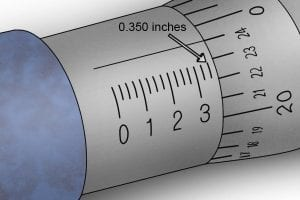The value of the first significant figure of a measurement can be found on the sleeve scale. On an imperial micrometer, this will be given in inches and tenths of an inch.

The smallest value that can be read from the sleeve scale is 0.025 inches.

The value on the sleeve scale is the number immediately to the left of the thimble.

In this instance, the value is 0.350 inches.

The value of the first significant figure of a measurement can be found on the sleeve scale. On an imperial micrometer, this will be given in inches and tenths of an inch.

The smallest value that can be read from the sleeve scale is 0.025 inches.The value on the sleeve scale is the number immediately to the left of the thimble.

In this instance, the value is 0.350 inches.### Step 2 - Look at thimble scale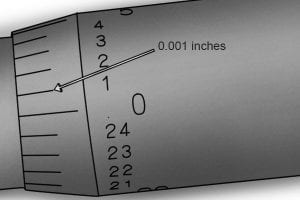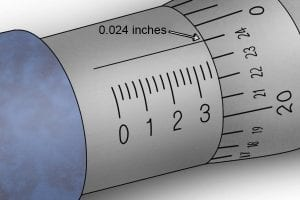Then, look at the thimble scale. The thimble scale of a metric micrometer has a measuring range of 0.025 inches (one complete revolution), and is divided into 25 increments. Each increment represents 0.001 inches.

When reading the thimble scale, identify the number that aligns best with the index line on the sleeve scale. If the index line falls between two values, the reading will be the number immediately below the index line.

If this is the case, you should estimate the final part of the measurement or, if your micrometer has one, consult the vernier scale. In the example, the value on the thimble scale is 0.024 inches.

Then, look at the thimble scale.

The thimble scale of a metric micrometer has a measuring range of 0.025 inches (one complete revolution), and is divided into 25 increments. Each increment represents 0.001 inches.

When reading the thimble scale, identify the number that aligns best with the index line on the sleeve scale.If the index line falls between two values, the reading will be the number immediately below the index line.

If this is the case, you should estimate the final part of the measurement or, if your micrometer has one, consult the vernier scale.

In the example, the value on the thimble scale is 0.024 inches.### Step 3 - Look at vernier scaleIf you have a vernier micrometer, you may next read the value indicated on the vernier scale (if you don’t have a vernier scale or require the accuracy, go to Step 4).

The vernier scale of an imperial micrometer has a measuring range of 0.001 inches and is graduated in 10 increments. Each increment represents 0.0001 inches.

The reading on the vernier scale is the value that aligns best with any increment on the thimble scale (in this image, 0.0009″).

If you have a vernier micrometer, you may next read the value indicated on the vernier scale (if you don’t have a vernier scale or require the accuracy, go to Step 4).

The vernier scale of an imperial micrometer has a measuring range of 0.001 inches and is graduated in 10 increments. Each increment represents 0.0001 inches.

The reading on the vernier scale is the value that aligns best with any increment on the thimble scale (in this image, 0.0009″).### Step 4 - Add values together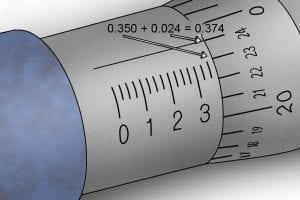To read the final measurement, add the values from each of the scales together (sleeve + thimble + vernier = measured value).

e.g. 0.350 + 0.024 = 0.374 inches (there is no vernier reading in this instance).

To read the final measurement, add the values from each of the scales together (sleeve + thimble + vernier = measured value).

e.g. 0.350 + 0.024 = 0.374 inches (there is no vernier reading in this instance).Call Now Button